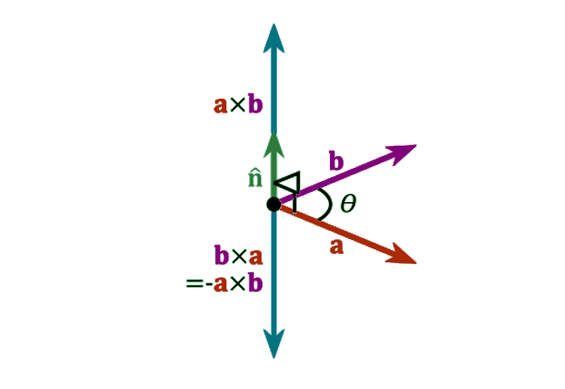# Cross Product Formula

The cross product or vector product is a binary operation on two vectors in three-dimensional space (R3) and is denoted by the symbol x. Two linearly independent vectors a and b, the cross product, a x b, is a vector that is perpendicular to both a and b and therefore normal to the plane containing them.Cross Product is given by,

$\LARGE A\times B=\begin{vmatrix} i & j & k\\ a_{1} & a_{2}& a_{3} \\ b_{1} & b_{2}& b_{3} \end{vmatrix}$

Where,
a1, a2, a3 are the components of the vector
$$\begin{array}{l}\overrightarrow{a}\end{array}$$
and b1, b2 and b3 are the components of
$$\begin{array}{l}\overrightarrow{b}\end{array}$$
.

Cross Product Formula is given by,

$\LARGE a\times b=\left | a \right |\left | b \right |\sin \theta$

Cross product formula is used to determine the cross product or angle between any two vectors based on the given problem.

### Solved Examples

Question 1:Calculate the cross products of vectors a = <3, 4, 7> and b = <4, 9, 2>.

Solution:

The given vectors are, a = (3, 4, 7) and b = (4, 9, 2)
The cross product is given by
$$\begin{array}{l}\times\end{array}$$
b =
$$\begin{array}{l}\begin{vmatrix} i & j & k\\ a_{1} & a_{2}& a_{3} \\ b_{1} & b_{2}& b_{3} \end{vmatrix}\end{array}$$

$$\begin{array}{l}\times\end{array}$$
b =
$$\begin{array}{l}\begin{vmatrix} i & j & k \\3 & 4 & 7 \\4 & 9 & 2 \end{vmatrix}\end{array}$$

$$\begin{array}{l}\times\end{array}$$
b =
$$\begin{array}{l}i(4\times 2-9\times 7)-j(3 \times 2 – 4\times 7)+k(3\times 9-4\times 4)\end{array}$$

a

$$\begin{array}{l}\times\end{array}$$
b =
$$\begin{array}{l}i(8-63)-j(6-28)+k(27-16)\end{array}$$

$$\begin{array}{l}\times\end{array}$$
b =
$$\begin{array}{l}-55i+22j+11k\end{array}$$

Question 2:

Find the angle between two vector a and b, where a =<-4, 3, 0> and b =<2, 0, 0>

Solution:

We know that, the formula to find the angle between two vectors is

Sin θ = a × b / |a| |b|

Therefore, θ = sin-1[a × b / |a| |b|]

Now, we have to find the cross product of two vectors and b:

$$\begin{array}{l}a\times b = \begin{vmatrix} i&j & k\\ -4 & 3 & 0\\ 2 & 0 & 0 \end{vmatrix}\end{array}$$

= i (0) -j(0) +k(-6)

a × b = -6k

$$\begin{array}{l}|a|= \sqrt{16+9 +0} = 5\end{array}$$

$$\begin{array}{l}|b|= \sqrt{4+0+0} = 2\end{array}$$

While finding the angle between two vectors, substitute the magnitude of the vector value, Thus,

|a × b| = 6

Therefore,  θ = sin-1[|a × b|/ |a| |b|]

θ = sin-1[ 6/ 5 .2 ]

θ = sin-1[ 3/ 5 ] = 36.87°

Hence, the angle between two vectors, a and b (θ) is 36.87°